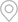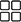当前位置：首页 > 新闻资讯 > 公司新讯### 新闻资讯热门推荐### 震惊！四川发电机厂家的柴油发电机组每小时油耗参考表泄露了1、燃油消耗率，不同品牌的柴油发电机组，其燃油消耗率不同，消耗油量就不同;

2、用电负载的大小，负载大了油门大耗油就大些，反之负载小了相对油耗也就耍小些。30kw柴油发电机组油耗量=6.3公斤(kg) =7.8升(L)

45kw柴油发电机组油耗量=9.45公斤(kg) =11.84升(L)

50kw柴油发电机组油耗量=10.5公斤(kg) =13. 1升(L)

75kw柴油发电机组油耗量=15.7公斤(kg) =19.7升(L)

100kw柴油发电机组油耗量=21公斤(kg) =26. 25升(L)

150kw柴油发电机组油耗量=31.5公斤(kg) =39. 4升(L)

200kw柴油发电机组油耗量=40公斤(kg) =50升(L)

250kw柴油发电机组油耗量=52.5公斤(kg) =65. 6升(L)

300kw柴油发电机组油耗量=63公斤(kg) =78. 75升(L)

350kw柴油发电机组油耗量=73.5公斤(kg) =91.8升(L)

400kw柴油发电机组油耗量=84.00公斤(kg) =105.00升(L)

450kw柴油发电机组油耗量=94.50公斤(kg) =118.00升(L)

500kw柴油发电机组油耗量=105.00公斤(kg) =131. 20升(L)